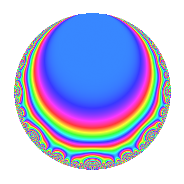Properties

 Label 164.2.aLevel 164 Weight 2 Character orbit a Rep. character $$\chi_{164}(1,\cdot)$$ Character field $$\Q$$ Dimension 4 Newforms 1 Sturm bound 42 Trace bound 0

Related objects

Defining parameters

 Level: $$N$$ = $$164 = 2^{2} \cdot 41$$ Weight: $$k$$ = $$2$$ Character orbit: $$[\chi]$$ = 164.a (trivial) Character field: $$\Q$$ Newforms: $$1$$ Sturm bound: $$42$$ Trace bound: $$0$$

Dimensions

The following table gives the dimensions of various subspaces of $$M_{2}(\Gamma_0(164))$$.

Total New Old
Modular forms 24 4 20
Cusp forms 19 4 15
Eisenstein series 5 0 5

The following table gives the dimensions of the cuspidal new subspaces with specified eigenvalues for the Atkin-Lehner operators and the Fricke involution.

$$2$$$$41$$FrickeDim.
$$-$$$$+$$$$-$$$$4$$
Plus space$$+$$$$0$$
Minus space$$-$$$$4$$

Trace form

 $$4q$$ $$\mathstrut +\mathstrut 2q^{3}$$ $$\mathstrut +\mathstrut 4q^{5}$$ $$\mathstrut +\mathstrut 12q^{9}$$ $$\mathstrut +\mathstrut O(q^{10})$$ $$4q$$ $$\mathstrut +\mathstrut 2q^{3}$$ $$\mathstrut +\mathstrut 4q^{5}$$ $$\mathstrut +\mathstrut 12q^{9}$$ $$\mathstrut +\mathstrut 4q^{11}$$ $$\mathstrut -\mathstrut 10q^{15}$$ $$\mathstrut -\mathstrut 4q^{17}$$ $$\mathstrut +\mathstrut 6q^{19}$$ $$\mathstrut -\mathstrut 12q^{23}$$ $$\mathstrut +\mathstrut 12q^{25}$$ $$\mathstrut -\mathstrut 10q^{27}$$ $$\mathstrut -\mathstrut 4q^{29}$$ $$\mathstrut -\mathstrut 8q^{31}$$ $$\mathstrut -\mathstrut 20q^{33}$$ $$\mathstrut -\mathstrut 26q^{35}$$ $$\mathstrut +\mathstrut 16q^{37}$$ $$\mathstrut -\mathstrut 24q^{39}$$ $$\mathstrut -\mathstrut 4q^{41}$$ $$\mathstrut +\mathstrut 4q^{43}$$ $$\mathstrut +\mathstrut 4q^{45}$$ $$\mathstrut -\mathstrut 6q^{47}$$ $$\mathstrut +\mathstrut 16q^{49}$$ $$\mathstrut -\mathstrut 4q^{51}$$ $$\mathstrut -\mathstrut 16q^{53}$$ $$\mathstrut -\mathstrut 2q^{55}$$ $$\mathstrut +\mathstrut 4q^{57}$$ $$\mathstrut +\mathstrut 12q^{59}$$ $$\mathstrut +\mathstrut 24q^{61}$$ $$\mathstrut -\mathstrut 10q^{63}$$ $$\mathstrut +\mathstrut 4q^{65}$$ $$\mathstrut +\mathstrut 28q^{67}$$ $$\mathstrut -\mathstrut 28q^{69}$$ $$\mathstrut -\mathstrut 2q^{71}$$ $$\mathstrut +\mathstrut 8q^{73}$$ $$\mathstrut +\mathstrut 30q^{75}$$ $$\mathstrut +\mathstrut 8q^{77}$$ $$\mathstrut -\mathstrut 18q^{79}$$ $$\mathstrut +\mathstrut 28q^{81}$$ $$\mathstrut -\mathstrut 12q^{83}$$ $$\mathstrut +\mathstrut 32q^{85}$$ $$\mathstrut +\mathstrut 44q^{87}$$ $$\mathstrut +\mathstrut 4q^{89}$$ $$\mathstrut +\mathstrut 36q^{91}$$ $$\mathstrut -\mathstrut 28q^{93}$$ $$\mathstrut +\mathstrut 14q^{95}$$ $$\mathstrut +\mathstrut 16q^{97}$$ $$\mathstrut +\mathstrut 58q^{99}$$ $$\mathstrut +\mathstrut O(q^{100})$$

Decomposition of $$S_{2}^{\mathrm{new}}(\Gamma_0(164))$$ into irreducible Hecke orbits

Label Dim. $$A$$ Field CM Traces A-L signs $q$-expansion
$$a_2$$ $$a_3$$ $$a_5$$ $$a_7$$ 2 41
164.2.a.a $$4$$ $$1.310$$ 4.4.25808.1 None $$0$$ $$2$$ $$4$$ $$0$$ $$-$$ $$+$$ $$q+(-\beta _{1}+\beta _{2})q^{3}+(2-\beta _{2}+\beta _{3})q^{5}+\cdots$$

Decomposition of $$S_{2}^{\mathrm{old}}(\Gamma_0(164))$$ into lower level spaces

$$S_{2}^{\mathrm{old}}(\Gamma_0(164)) \cong$$ $$S_{2}^{\mathrm{new}}(\Gamma_0(41))$$$$^{\oplus 3}$$$$\oplus$$$$S_{2}^{\mathrm{new}}(\Gamma_0(82))$$$$^{\oplus 2}$$